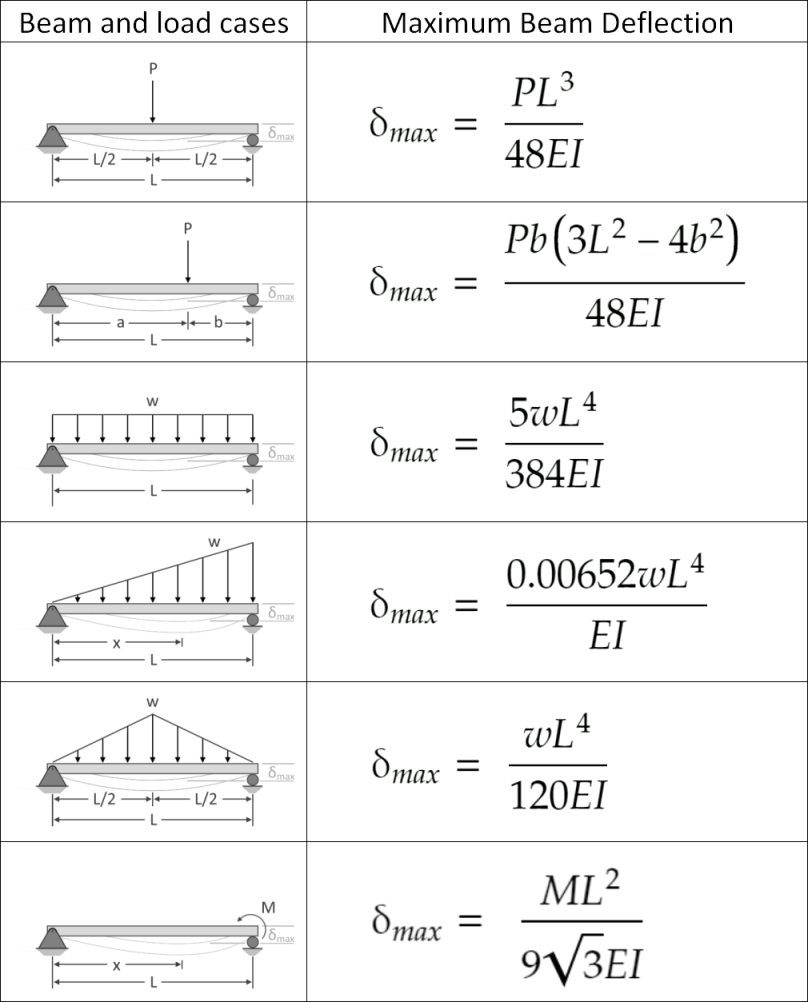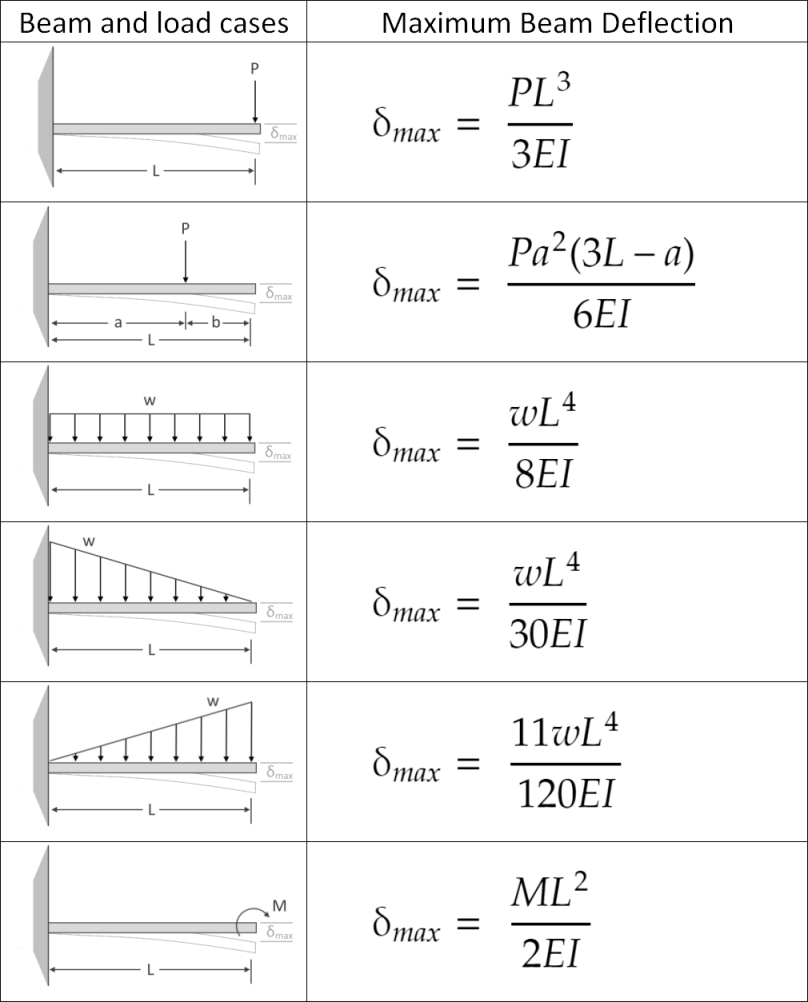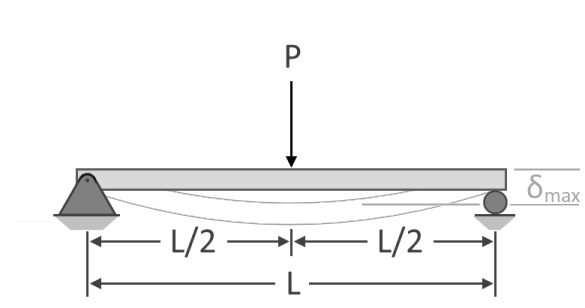# Beam Deflection Calculator

Created by Nicholas Swanson and Kenneth Alambra
Reviewed by Bogna Szyk
Last updated: Jun 17, 2022

This beam deflection calculator will help you determine the maximum beam deflection of simply-supported beams, and cantilever beams carrying simple load configurations. You can choose from a selection of load types that can act on any length of beam you want. The magnitude and location of these loads affect how much the beam bends. In this beam deflection calculator, you'll learn about the different beam deflection formulas used to calculate simply-supported beam deflections and cantilever beam deflections. You will also learn how the beam's modulus of elasticity and its cross-sectional moment of inertia affect the calculated maximum beam deflection.

Beam deflection is an important part of beam analysis, but another important part is stress analysis. A powerful tool to study beam bending stresses is the section modulus, which you can calculate using our section modulus calculator.

## What is beam deflection and beam bendingIn building construction, we usually use framing structures that are held in place by the foundations in the ground. These framing structures are like the skeletons of buildings, houses, and even bridges. In a frame, we call the vertical framing columns, and the horizontal ones beams. Beams are the long members of a structure that carry the loads brought by the horizontal slabs of the structures like solid concrete floors, wooden floor joist systems, and roofs.

When beams carry loads that are too heavy for them, they start to bend. We call the amount of beam bending beam deflection. Beam deflection is the vertical displacement of a point along the centroid of a beam. We can also consider the beam's surface as our reference point, as long as there are no changes in the beam's height or depth during the bending.

## How to calculate the maximum beam deflection

We equipped our beam deflection calculator with the formulas that engineers and engineering students use to quickly determine the maximum deflection a specific beam will experience due to the load it carries. However, these formulas can only solve simple loads and a combination of these loads. We have tabulated these formulas for you, as shown below:

Simply-supported beam deflection formulasCantilever beam deflection formulas## Method of superposition

To calculate the maximum deflection of a beam with a combination of loads, we can use the method of superposition. The method of superposition states that we can approximate a beam's total deflection by adding together all the deflections brought about by each load configuration. However, this method only gives us an approximate value for the actual maximum deflection. Calculating complicated loads would require us to use what is known as the double integration method.

## Stiffness of the beam

Calculating beam deflection requires knowing the stiffness of the beam and the amount of force or load that would influence the bending of the beam. We can define the stiffness of the beam by multiplying the beam's modulus of elasticity, E, by its moment of inertia, I. The modulus of elasticity depends on the beam's material. The higher a material's modulus of elasticity, the more a deflection can sustain enormous loads before it reaches its breaking point. Concrete's modulus of elasticity is between 15-50 GPa (gigapascals), while steel's tends to be around 200 GPa and above. This difference in the values of modulus of elasticity shows that concrete can only withstand a small amount of deflection and will experience cracking sooner than steel.

You can learn more about the modulus of elasticity by checking out our stress calculator. On the other hand, to determine the moment of inertia for a particular beam cross-section, you can visit our moment of inertia calculator. The moment of inertia represents the amount of resistance a material has to rotational motion. The moment of inertia depends on the dimensions of the material's cross-section.

The moment of inertia also varies depending on which axis the material is rotating along. To further understand this concept, let us consider the cross-section of a rectangular beam with a width of 20 cm and a height of 30 cm. Using the formulas that you can also see in our moment of inertia calculator, we can calculate the values for the moment of inertia of this beam cross-section as follows:

Iₓ = width × height³ / 12
= 20 × (30³)/12
= 45,000 cm⁴

Iᵧ = height × width³ / 12
= 30 × (20³)/12
= 20,000 cm⁴

Notice how there are two values for the moment of inertia. That's because we can consider the beam to bend vertically along the beam span (or experience a bending moment around the x-axis) and laterally along the beam span (or bend around the y-axis). Since we are considering the deflection of the beam when it bends vertically or around the x-axis, we have to use Iₓ for our computations. The moment of inertia values we obtained tell us that the beam is harder to bend with a vertical load and easier to bend if subjected to a horizontal lateral load. This difference in the moment of inertia values is the reason why we see beams in this configuration - wherein their height is greater than their width.

## Understanding the beam deflection formulas

Now that we know the concepts of modulus of elasticity and moment of inertia, we can now understand why these variables are the denominators in our beam deflection formulas. The formulas show that the stiffer the beam is, the smaller its deflection will be. However, by inspecting our formulas, we can also say that the beam's length also directly affects the deflection of the beam. The longer the beam gets, the more that it can bend, and the greater the deflection can be.

Loads, on the other hand, affect the beam's deflection in two ways: the direction of the deflection and the magnitude of the deflection. Downward loads tend to deflect the beam downwards. Loads can be in the form of a single point load, linear pressure, or moment load. The formulas in this calculator only focus on either the downward or upward directions for the point load and distributed loads. Distributed loads are similar to pressure, but only consider the length of the beam and not the width of the beam. The formulas in this calculator also consider the moment or torque load, either in a clockwise or counterclockwise direction. Just consult the directions of the arrows in the formula's corresponding image to figure out which directions has a positive load value.

## Sample beam deflection calculationFor a sample calculation of beam deflection, let us consider a simple wooden bench with legs 1.5 meters apart from each other at their centers. Let us say we have a 4-cm thick, 30-cm wide eastern white pine plank that acts as the seat for this bench. We can consider this seat as a beam that will deflect whenever someone sits on the bench. Given the dimensions of this seat, we can then calculate its moment of inertia, similar to our example above. Since we need to calculate Iₓ, its moment of inertia would be:

Iₓ = width * height³ / 12
= 30*(4³)/12
= 160.0 cm⁴ or 1.6x10⁻⁶ m⁴

Eastern white pine has a modulus of elasticity of 6,800 MPa (6.8x10⁹ Pa), which is the value we obtained from the . You can also easily obtain the value of the modulus of elasticity for other materials, such as steel and concrete, from the internet or from your local library. Now that we know these values, let us consider the load that this bench will carry. Let's suppose that a 400 N child sits in the middle of the bench. We can now calculate the deflection the bench's seat will experience due to a point load at its center:

δₘₐₓ = P * L³ / (48 * E * I)
δₘₐₓ = (400 N) * (1.5 m)³ / (48 * 6.8x10⁹ Pa * 1.6x10⁻⁶ m⁴)
δₘₐₓ = 0.002585 m = 2.5850 mm

This means that the bench seat will sag around 2.6 millimeters from its original position when the child sits in the middle of the bench.

If you found this topic interesting and would love to learn more about the strength of materials, you might like our factor safety calculator as well. You can also check out our force converter if you want to explore the different units used in point loads and in calculating forces.

Nicholas Swanson and Kenneth Alambra
Beam type
Simply-supported beamInput Values
Span length, L
ft
kip
Modulus of Elasticity, E
psi
Moment of Inertia, Ix
in⁴
Stiffness of the beam, EIx
MNm²
Output value
Maximum deflection, δmax
in
People also viewed…

### Board foot

Board foot calculator determines the volume of lumber in board footage.

### Circumference

Use this free circumference calculator to find the area, circumference and diameter of a circle.

### Millionaire

This millionaire calculator will help you determine how long it will take for you to reach a 7-figure saving or any financial goal you have. You can use this calculator even if you are just starting to save or even if you already have savings.

### Rip rap

Find the average diameter of rocks to use for your embankment with this rip rap calculator.